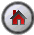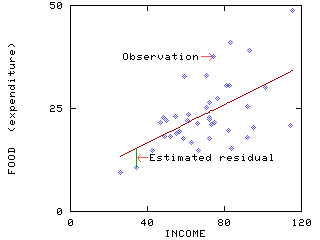Ordinary Least Squares

## Ordinary Least Squares Regression

The `OLS` command will estimate the parameters of a linear regression equation by the method of ordinary least squares. The general command format is:

 `OLS depvar indeps / options `

where depvar is the dependent variable, indeps is a list of the explanatory variables and options is a list of desired options. There are many useful options on the `OLS` command and some of these will be illustrated in this guide.

#### Appendixes[SHAZAM Guide home]

### 2-variable Regression Analysis

This example uses the Griffiths, Hill and Judge data set on household expenditure for food. Consider a simple linear regression with `FOOD` as the dependent variable and `INCOME` as the explanatory variable. The following SHAZAM program reads the data from the file `GHJ.txt`, assigns variable names and runs the regression. Note that the `READ` command assumes that the data file is in the current directory (or folder).

 ```SAMPLE 1 40 READ (GHJ.txt) FOOD INCOME OLS FOOD INCOME STOP ```

The output file of results follows.

 ``` |_SAMPLE 1 40 |_READ (GHJ.txt) FOOD INCOME UNIT 88 IS NOW ASSIGNED TO: GHJ.txt 2 VARIABLES AND 40 OBSERVATIONS STARTING AT OBS 1 |_OLS FOOD INCOME OLS ESTIMATION 40 OBSERVATIONS DEPENDENT VARIABLE = FOOD ...NOTE..SAMPLE RANGE SET TO: 1, 40 R-SQUARE = .3171 R-SQUARE ADJUSTED = .2991 VARIANCE OF THE ESTIMATE-SIGMA**2 = 46.853 STANDARD ERROR OF THE ESTIMATE-SIGMA = 6.8449 SUM OF SQUARED ERRORS-SSE= 1780.4 MEAN OF DEPENDENT VARIABLE = 23.595 LOG OF THE LIKELIHOOD FUNCTION = -132.672 VARIABLE ESTIMATED STANDARD T-RATIO PARTIAL STANDARDIZED ELASTICITY NAME COEFFICIENT ERROR 38 DF P-VALUE CORR. COEFFICIENT AT MEANS INCOME .23225 .5529E-01 4.200 .000 .563 .5631 .6871 CONSTANT 7.3832 4.008 1.842 .073 .286 .0000 .3129 |_STOP ```

SHAZAM automatically includes an intercept coefficient in the regression and this is given the name `CONSTANT`. On the SHAZAM output, the intercept estimate is listed as the final coefficient estimate.

The results show that the estimated coefficient on `INCOME` (the slope coefficient) is `0.23225` and the intercept estimate is `7.3832`. The estimated equation can be written as:

```               FOOD = 7.38 + 0.232 INCOME + ê
```

where ê is the estimated residual. The figure below shows a scatterplot of the observations and the estimated regression line. (This figure corresponds to Figure 5.9 of Griffiths, Hill and Judge [1993, p. 187]).The `LIST` option on the `OLS` command will give more extensive output that includes a listing of the estimated residuals and the predicted values for the dependent variable. The use of the `LIST` option is shown with the SHAZAM command:

 `OLS FOOD INCOME / LIST `

The interested reader can look at the SHAZAM output generated with the `LIST` option.

#### Interpreting t-ratios

The OLS estimation results report the `ESTIMATED COEFFICIENT` and the estimated `STANDARD ERROR`. With the assumption that the errors are normally distributed these estimates can be used for hypothesis testing purposes. In the above example, a useful question to ask is: Is the estimated coefficient on `INCOME` significantly different from zero ? That is, does household income have an effect on the level of household expenditure for food ? To help answer this question the SHAZAM output reports the test statistic:

```         T-RATIO = ESTIMATED COEFFICIENT / STANDARD ERROR
```

The estimated coefficient is significantly different from zero (that is, the null hypothesis of a zero coefficient is rejected) if the t-ratio is "relatively large". The critical value is obtained from tables for the t-distribution with `N-K` degrees of freedom (`N` is the number of observations and `K` is the number of estimated coefficients). These tables are usually printed in the appendix to econometrics textbooks.

For the household food expenditure example the reported t-ratio for the coefficient on `INCOME` is `4.20`. The number of observations is 40 and the number of estimated coefficients is 2 and so the degrees of freedom (`DF`) is 38. By choosing a signficance level of 5% and considering a two-sided test (so that the critical region in each tail is 2.5%) the critical value obtained from printed tables is `2.024`. (Note that this critical value was approximated using the tabulated values for 30 and 40 degrees of freedom that are reported in the tables.) In absolute value, the t-ratio exceeds this critical value. Therefore, there is strong evidence to conclude that the estimated coefficient on `INCOME` is significantly different from zero.

#### Interpreting p-values

When interpreting t-ratios it can be inconvenient to consult statistical tables. To assist the user, SHAZAM reports the `P-VALUE` on the OLS estimation output. This value is computed as the tail probability for a two-tail test of the null hypothesis that the coefficient is 0. This is the probability of a Type I error - the probability of rejecting a true hypothesis.

The null hypothesis is rejected if the p-value is "small" (say smaller than 0.10, 0.05 or 0.01). For example, if the p-value is 0.078, this means that the null hypothesis cannot be rejected at a 5% significance level but can be rejected at a 10% significance level.

Note: SHAZAM only reports three decimal places for the p-value. So a value that is reported as `.000` actually means a value less than `.0005`. This can be interpreted as meaning that the null hypothesis of a zero coefficient is rejected at any reasonable significance level.

It is possible to use SHAZAM commands to compute p-values for test statistics.

#### Interpreting elasticities

For the household food expenditure relationship the estimated coefficient on `INCOME` measures the marginal effect. This gives the amount by which `FOOD` changes in response to a one unit change in `INCOME`.

Another measure of interest to economists is elasticity. This gives the percentage change in the dependent variable that results from a 1% change in the explanatory variable. The final column on the SHAZAM OLS estimation output reports the `ELASTICITY AT MEANS`.

For the example illustrated here, let `B1` be the estimated coefficient on `INCOME` and let `CM` and `PM` be the sample means of `FOOD` and `INCOME` respectively. The income elasticity evaluated at the sample means is computed as:

```         B1 (PM/CM) =  0.6871
```

When interpreting the meaning of the estimated coefficients and the elasticities users should take careful note of the units of measurement of the variables in the regression equation.

#### The LIST option

The SHAZAM output that follows shows the use of the `LIST` option on the `OLS` command.

 ``` |_OLS FOOD INCOME / LIST OLS ESTIMATION 40 OBSERVATIONS DEPENDENT VARIABLE = FOOD ...NOTE..SAMPLE RANGE SET TO: 1, 40 R-SQUARE = .3171 R-SQUARE ADJUSTED = .2991 VARIANCE OF THE ESTIMATE-SIGMA**2 = 46.853 STANDARD ERROR OF THE ESTIMATE-SIGMA = 6.8449 SUM OF SQUARED ERRORS-SSE= 1780.4 MEAN OF DEPENDENT VARIABLE = 23.595 LOG OF THE LIKELIHOOD FUNCTION = -132.672 VARIABLE ESTIMATED STANDARD T-RATIO PARTIAL STANDARDIZED ELASTICITY NAME COEFFICIENT ERROR 38 DF P-VALUE CORR. COEFFICIENT AT MEANS INCOME .23225 .5529E-01 4.200 .000 .563 .5631 .6871 CONSTANT 7.3832 4.008 1.842 .073 .286 .0000 .3129 OBS. OBSERVED PREDICTED CALCULATED NO. VALUE VALUE RESIDUAL 1 9.4600 13.382 -3.9223 * I 2 10.560 15.352 -4.7918 * I 3 14.810 17.254 -2.4440 * I 4 21.710 18.241 3.4689 I * 5 22.790 18.599 4.1913 I * 6 18.190 18.710 -.52021 * 7 22.000 18.915 3.0854 I * 8 18.120 19.446 -1.3265 *I 9 23.130 20.002 3.1285 I * 10 19.000 20.127 -1.1270 *I 11 19.460 20.496 -1.0362 *I 12 17.830 21.047 -3.2167 * I 13 32.810 21.116 11.694 I * 14 22.130 21.488 .64204 * 15 23.460 21.579 1.8815 I* 16 16.810 22.038 -5.2284 * I 17 21.350 22.703 -1.3526 *I 18 14.870 22.805 -7.9348 * I 19 33.000 23.738 9.2615 I * 20 25.190 23.752 1.4376 I* 21 17.770 24.101 -6.3308 * I 22 22.440 24.105 -1.6655 *I 23 22.870 24.159 -1.2889 *I 24 26.520 24.159 2.3611 I * 25 21.000 24.440 -3.4399 * I 26 37.520 24.628 12.892 I * 27 21.690 24.749 -3.0588 * I 28 27.400 25.111 2.2889 I * 29 30.690 26.200 4.4896 I * 30 19.560 26.393 -6.8332 * I 31 30.580 26.558 4.0219 I * 32 41.120 26.737 14.383 I * 33 15.380 26.753 -11.373 * I 34 17.870 28.706 -10.836 * I 35 25.540 28.706 -3.1664 * I 36 39.000 28.973 10.027 I * 37 20.440 29.487 -9.0468 * I 38 30.100 30.934 -.83371 *I 39 20.900 33.890 -12.990 * I 40 48.710 34.199 14.511 I * DURBIN-WATSON = 2.3703 VON NEUMANN RATIO = 2.4310 RHO = -.28193 RESIDUAL SUM = -.36060E-12 RESIDUAL VARIANCE = 46.853 SUM OF ABSOLUTE ERRORS= 207.53 R-SQUARE BETWEEN OBSERVED AND PREDICTED = .3171 RUNS TEST: 22 RUNS, 17 POS, 0 ZERO, 23 NEG NORMAL STATISTIC = .4755 |_STOP ```

The `LIST` option displays a table of results that contains the following:

 ` OBSERVED VALUE ` The observed value of the dependent variable. ` PREDICTED VALUE` The predicted value (also called estimated value or fitted value) of the dependent variable. `CALCULATED RESIDUAL   ` The difference between the observed and predicted values.

The right hand side of the output displays a rough plot of the residuals.

A property of ordinary least squares regression (when an intercept is included) is that the sum of the estimated residuals (and hence the mean of the estimated residuals) is 0. Note that the final part of the SHAZAM output reports:

``` RESIDUAL SUM =  -.36060E-12
```

That is, SHAZAM computes the sum of residuals as `.00000000000036060`. This shows that computer calculations can have some imprecision. Different computers may have numerical differences in the reporting of this result.